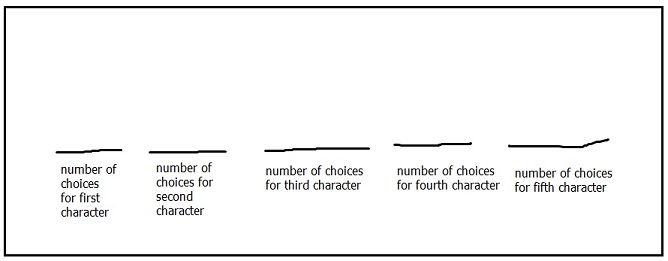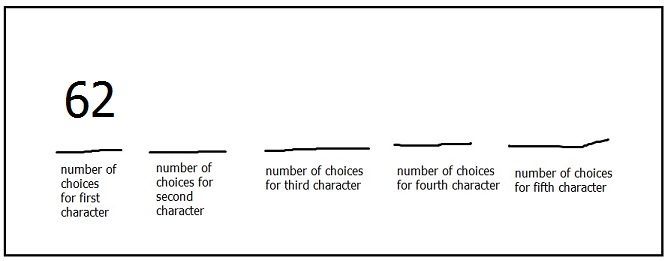# Counting with the multiplication rule

Counting is a really tough area of mathematics, but is also really important for understanding real life applications and, later, for finding probabilities. In this article, we will study one particular method used in counting: the multiplication rule.

## The multiplication rule

Imagine you are trying to guess someone’s password. If you know that the password is made up of 5 letters or numbers, we can imagine that you simply guess all 5 at once. But, we could also imagine that you guess the first letter or number, then the second, then the third, and so on. In the end, the result is the same but the way we think about it is different.

If you can think of a process in steps like this, then we can apply the multiplication rule. In order to find the total number of possible passwords, we first think of how many possibilities there are for the first character, how many for the second, and so on. We then multiply these to find the total number of possibilities.

A password is 5 characters long and is made up of letters and numbers. How many different passwords like this are possible?

We will think about the number of possibilities for each character individually.• There are 26 letters in the alphabet.
• There are 10 possible numbers for any for any character: 0, 1, 2, 3, 4, 5, 6, 7, 8, 9
• In a password, uppercase and lowercase letters are considered different, so there are 26 + 26 = 52 possible letters for any character.

For the first character, this means there are a total of 10 + 52 = 62 possibilities.For the next character, we still have 62 possibilities. There was no indication that the password can’t use the same letter or number twice. In fact, there are the same number of possibilities for each character. Filling this in and applying the multiplication rule we have:A password is 5 characters long, is made up of letters and numbers, and has no repeated characters. How many different passwords like this are possible?

This question is almost identical to the first one, except for one part: no repeated characters. Pay attention to wording like this!!

This tells you that after you have used one number or letter, you can’t use it again. So the number of choices for the first character is still 62. But now, you have used 1 of the characters, so there are only 61 to choose from for the next. This pattern continues after each choice is made.